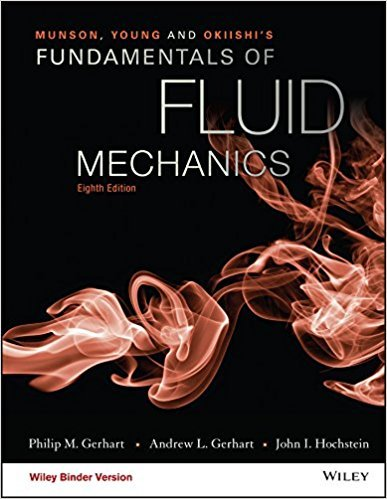×
Get Full Access to Fundamentals Of Fluid Mechanics - 8 Edition - Chapter 4.1 - Problem 4.5
Get Full Access to Fundamentals Of Fluid Mechanics - 8 Edition - Chapter 4.1 - Problem 4.5

×

# Streamlines are given in Cartesian coordinates by the equation. = U(y yx2 + y2).x2 + y2ISBN: 9781119080701 456

## Solution for problem 4.5 Chapter 4.1

Fundamentals of Fluid Mechanics | 8th Edition

• Textbook Solutions
• 2901 Step-by-step solutions solved by professors and subject experts
• Get 24/7 help from StudySoup virtual teaching assistantsFundamentals of Fluid Mechanics | 8th Edition

4 5 1 273 Reviews
25
2
Problem 4.5

Streamlines are given in Cartesian coordinates by the equation. = U(y yx2 + y2).x2 + y2 1.Plot the streamlines for = 0, 60.0625, and describe the physicalsituation represented by this equation. The parameter U is anupstream uniform velocity of 1.0 ft/s.

Step-by-Step Solution:
Step 1 of 3

o rl((ulc{Til[eJr,*-ft1rrutl 2 rYriiet\^te.] \.,: l- l-l--F i c\rpo\q-- C\rp\t- r.fi'\Uci\$\rl{orcu i. * Iaorccsn ,Boncl tnc{ crhmg) - c,F-trtrct1rg1.'a,rqrs C,r1al$$*6lnc\ tL i^t(caE{run J\ \t : O *p \nKrc Step 2 of 3 ###### Chapter 4.1, Problem 4.5 is Solved Step 3 of 3 ##### Textbook: Fundamentals of Fluid Mechanics ##### Edition: 8 ##### Author: Philip M. Gerhart, Andrew L. Gerhart, John I. Hochstein ##### ISBN: 9781119080701 This full solution covers the following key subjects: . This expansive textbook survival guide covers 112 chapters, and 1357 solutions. Fundamentals of Fluid Mechanics was written by and is associated to the ISBN: 9781119080701. Since the solution to 4.5 from 4.1 chapter was answered, more than 247 students have viewed the full step-by-step answer. This textbook survival guide was created for the textbook: Fundamentals of Fluid Mechanics, edition: 8. The answer to “Streamlines are given in Cartesian coordinates by the equation. = U(y yx2 + y2).x2 + y2 1.Plot the streamlines for = 0, 60.0625, and describe the physicalsituation represented by this equation. The parameter U is anupstream uniform velocity of 1.0 ft/s.” is broken down into a number of easy to follow steps, and 41 words. The full step-by-step solution to problem: 4.5 from chapter: 4.1 was answered by , our top Science solution expert on 03/16/18, 03:21PM. ## Discover and learn what students are asking Calculus: Early Transcendental Functions : Graphs and Models ?In Exercises 5-14, sketch the graph of the equation by point plotting. \(y=(x-3)^{2}$$

Calculus: Early Transcendental Functions : Evaluating Limits Analytically
?Finding a Limit In Exercises 5-36, find the limit. $$\lim _{x \rightarrow-3} x^{4}$$

Statistics: Informed Decisions Using Data : Goodness-of-Fit Test
?Explain why chi-square goodness-of-fit tests are always right tailed.

Unlock Textbook Solution